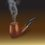# Reversing Real Analysis

We all know that the whole study of real analysis is based on the two special properties of the field of reals, viz., The Order Completeness Property (which ensures that there is no gap in the real line), and The Archimedean Property (which ensures the existence and unboundedness of the natural numbers). These two properties are independent and somewhat axiomatic in the study of real analysis. However, many beginner-level books on the subject consider the Least Upper Bound Property to be synonymous to the Order Completeness Property of the reals, and deduce thence the other properties of reals like the Nested Interval Property, the Bolzano-Weierstrass Property, the Monotone Convergence Theorem of Sequences, the Cauchy Principle of Convergence of Sequences, etc. However, the actual picture is not so. If we consider the Archimedean Property separately as an axiomatic property of the reals, we can establish the equivalence of all the aforementioned properties of reals, all of which are nothing but the Order Completeness Property of reals in disguise. For example, in textbooks we see that they have deduced the Nested Interval Property of Reals considering the Least Upper Bound Property (L.U.B.) and the Archimedean Property as axioms. But we can go the other way round. We can consider the Nested Interval Property (N.I.P.) and the Archimedean Property of Reals as axioms and prove the validity of the Least Upper Bound Property. All undergraduates here, try this as an exercise. I will upload my paper that addresses this problem (of proving the L.U.B. property from the N.I.P. and the Archimedean property) in a few days.Note by Kuldeep Guha Mazumder
6 years ago

This discussion board is a place to discuss our Daily Challenges and the math and science related to those challenges. Explanations are more than just a solution — they should explain the steps and thinking strategies that you used to obtain the solution. Comments should further the discussion of math and science.

When posting on Brilliant:

• Use the emojis to react to an explanation, whether you're congratulating a job well done , or just really confused .
• Ask specific questions about the challenge or the steps in somebody's explanation. Well-posed questions can add a lot to the discussion, but posting "I don't understand!" doesn't help anyone.
• Try to contribute something new to the discussion, whether it is an extension, generalization or other idea related to the challenge.

MarkdownAppears as
*italics* or _italics_ italics
**bold** or __bold__ bold
- bulleted- list
• bulleted
• list
1. numbered2. list
1. numbered
2. list
Note: you must add a full line of space before and after lists for them to show up correctly
paragraph 1paragraph 2

paragraph 1

paragraph 2

[example link](https://brilliant.org)example link
> This is a quote
This is a quote
    # I indented these lines
# 4 spaces, and now they show
# up as a code block.

print "hello world"
# I indented these lines
# 4 spaces, and now they show
# up as a code block.

print "hello world"
MathAppears as
Remember to wrap math in $$ ... $$ or $ ... $ to ensure proper formatting.
2 \times 3 $2 \times 3$
2^{34} $2^{34}$
a_{i-1} $a_{i-1}$
\frac{2}{3} $\frac{2}{3}$
\sqrt{2} $\sqrt{2}$
\sum_{i=1}^3 $\sum_{i=1}^3$
\sin \theta $\sin \theta$
\boxed{123} $\boxed{123}$